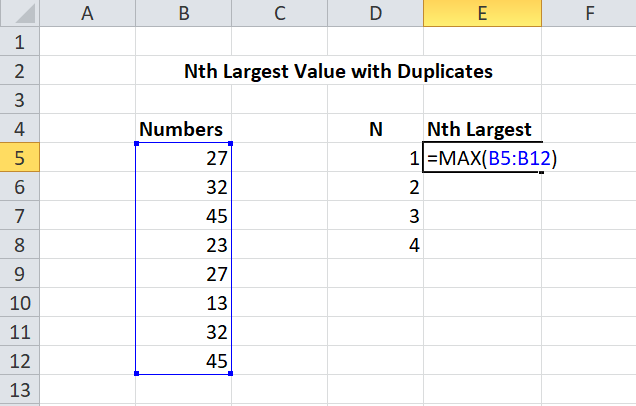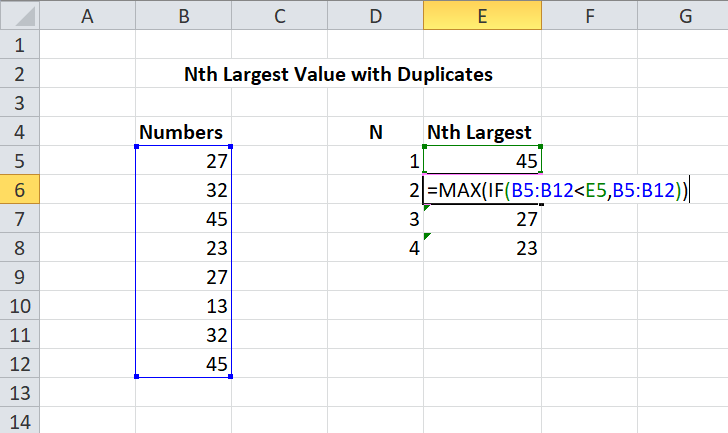Open in App
Not now

# How to Find the Nth Largest Value With Duplicates in Excel?

• Last Updated : 13 Oct, 2021

In Microsoft Excel, in order to find Nth Largest value (with duplicates), an array formula based on MAX and IF functions is used. First, we find the largest value of range using the MAX function. In this case, the range is from B5 to B12.

`= MAX(range)      `Let the cell having the largest value be E5 i.e. 45. Once we find the largest value, we simply use the formula given below to check all values in the taken range against the “last largest value”. As this is an array formula, we must enter it with Ctrl+Shift+Enter.

``` = MAX(IF(range < E5, range))

range: Range of cells ```Explanation: After applying the above formula, values lower than the last largest remain the same but any value greater than the last largest becomes FALSE. As the formula gets copied down the column, each row (in this case E5) increments, hence MAX and IF return a new array, forming the Nth largest number series.

Note: LARGE function is not used as it returns duplicate values if they exist in data.

My Personal Notes arrow_drop_up